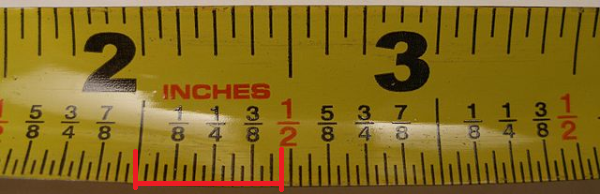# How to Read a Tape Measure

## Understanding Tape Measures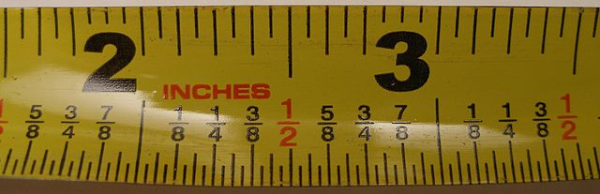A tape measure, also called measuring tape, is a type of flexible ruler. Tape measures are made from a variety of materials, including fiber glass, plastic and cloth. They are among the most common measuring tools used today.

Generally speaking, the term “tape measure” refers to a roll-up, self-retracting style tape measure that’s designed for carpentry. The actual tape potion of the measure, called the ‘ribbon,’ is usually constructed from a stiff metallic material that can stiffen when needed but can also roll up for simple use and storage.  However, the term covers all types of tape measures – even tailor’s tape.

The modern idea of tape measures originated in tailoring with cloth tape used to alter or fix clothing. It wasn’t until carpenters adopted the Farrand Rapid Rule, patented by Hiram Farrand, that the tape measure became a staple of common usage. Ferrand’s design was later sold to Stanley Works.

Tape measures come in both Imperial Units (inches and feet) and metric (centimeters and meters), featuring unit breakdowns for further accuracy. Specialized versions can include markings that cover truss lengths for roofing and stud intervals for housing.

The guides below for how to read a tape measure and how to measure on a tape measure assume Imperial (American) units for the explanations.

##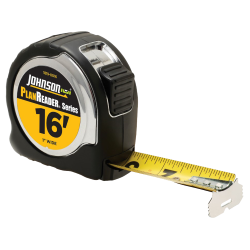How to Read a Tape Measure

1. Find/read the markings. On a standard tape measure, the biggest marking is the inch mark (which generally has the biggest number, if it has them).
2. As the increments decrease, so does the length of the mark. For example, ½" has a bigger mark than ¼" which has a bigger mark than ⅛", and so on.3. Read 1 inch. The space from the largest mark to another is 1 inch.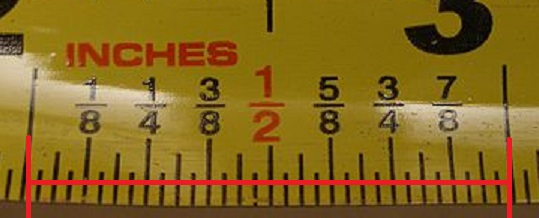4. Read one-half inch. Same principle as reading one inch, only this time the space between the second-biggest mark and the biggest is read. You can think of a half-inch mark as half way between a full inch.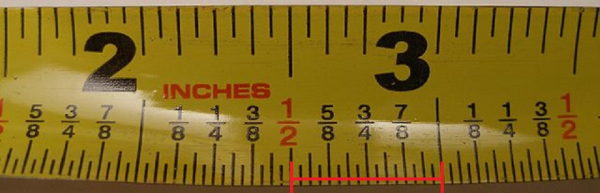5. The remaining markings follow a similar pattern. ¼" is half of ½". ⅛" is half of ¼". Most tape measure markings go as small as 116;". This tape divides one more time, down to 132".

##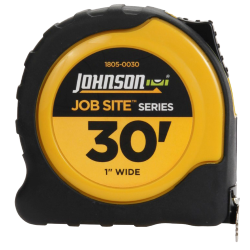How to Measure Using a Tape Measure

1. Measure a length. Put the end of the measure at one end of the item or space you want to measure. When the length stops, take a reading on the tape measure.
2. Find the length. In order to determine the length, you must add the lengths between inches together. For example, the image below has a measurement that goes beyond the space between two inch marks (that is, one full inch). In order to find the length, add the length of the inch (1) with the space between the second inch mark and the third. In this case, you’d add 1 inch + 1/4 inch to get 1¼ inch, or “one and a quarter inches.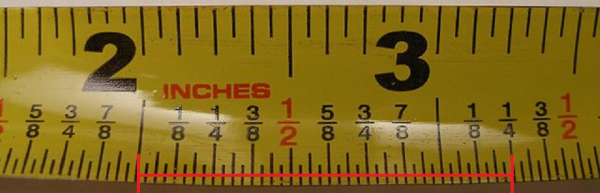3. For a length less than 1 inch, simply read off the tape measure the length. If the increments of an inch are not labeled, determine the marking’s increment and add together the respective fractions.
4. As an example, the image below shows a length that goes from the inch mark to an unlabeled marking. We know it’s more than 3/4 of an inch and less than one full inch. The marking is half way between 3/4 (6/8) and 7/8. Therefore, the marking is half of 1/8, or 1/16. Taking this knowledge, you simply add the known fractions to find the length. Convert 3/4 to 12/16 for common denominators and add 12/16 + 1/16 to get 13/16 – that’s your length.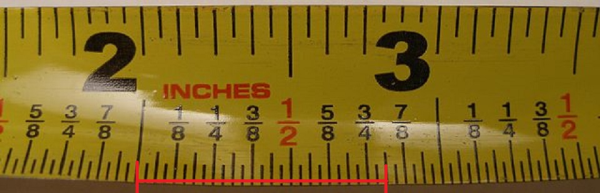## Tape Measure Test

Put your newfound skills to the test! What is the measurement over the range marked by the red lines? Use every technique at your disposal to come up with the answer as quickly as you can.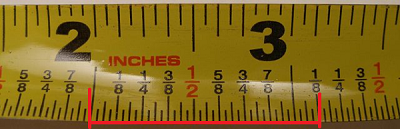Let's start simple. The distance from the 2" to 3" marker is, of course, 1". From the inch mark to the ⅛" mark is ⅛". 1" + ⅛" = 1 ⅛".A little more difficult. Break it down into simpler parts. Think of this as what's halfway between 58" and 34"? Find the lowest common denominator: 58" and 68". To find the middle of these two numbers, multiply the denominator by 2. 1016" and 1216". Halfway between these two numbers? 1116".